8.3 Justify that the following reactions are redox reactions:

(a) CuO(s) + H2(g) Cu(s) + H2O(g)

(b) Fe2O3(s) + 3CO(g) 2Fe(s) + 3CO2(g)

(c) 4BCl3(g) + 3LiAlH4(s) 2B2H6(g) + 3LiCl(s) + 3 AlCl3 (s)

(d) 2K(s) + F2(g) 2K+F (s)

(e) 4 NH3(g) + 5 O2(g) 4NO(g) + 6H2O(g)

(a)

Let us write the oxidation number of each element involved in the given reaction as:

Here, the oxidation number of Cu decreases from +2 in  to 0 in  i.e.,  is reduced to . A/so, the oxidation number of  increases from 0 in  to +1 in i.e.,  is oxidized to O. Hence, this reaction is a redox reaction.

(b)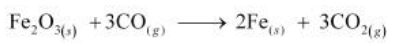Let us write the oxidation number of each element in the given reaction as: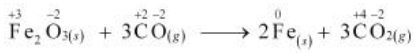Here, the oxidation number of Fe decreases from +3 in  to 0 in  i.e.,  is reduced to . On the other hand, the oxidation number of  increases from +2 in  to +4 in  i.e.,  is oxidized to . Hence, the given raaction is a redox reaction.

(c)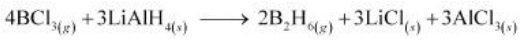The oxidation number of each element in the given reaction can be represented as: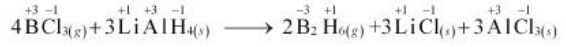In this reaction, the oxidation number of B decreases from +3 in BCl to –3 in BH${}_{6}$. i.e., BCl is reduced to BH${}_{6}$. Also, the oxidation number of H increases from –1 in LiAlH${}_{4}$ to +1 in BH${}_{6}$ i.e., LiAlH4 is oxidized to BH${}_{6}$. Hence, the given reaction is a redox reaction.

(d)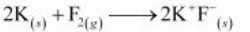The oxidation number of each element in the given reaction can be represented as: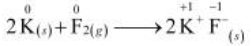In this reaction, the oxidation number of K increases from 0 in K to +1 in KF i.e., K is oxidized to KF. On the other hand, the oxidation number of F decreases from 0 in F${}_{2}$ to –1 in KF i.e., F is reduced to KF.

Hence, the above reaction is a redox reaction.

(e)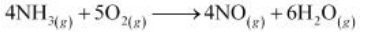The oxidation number of each element in the given reaction can be represented as: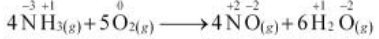Here, the oxidation number of N increases from –3 in NH to +2 in NO. On the other hand, the oxidation number of O decreases from 0 in O to –2 in NO and HO i.e., O is reduced. Hence, the given reaction is a redox reaction.